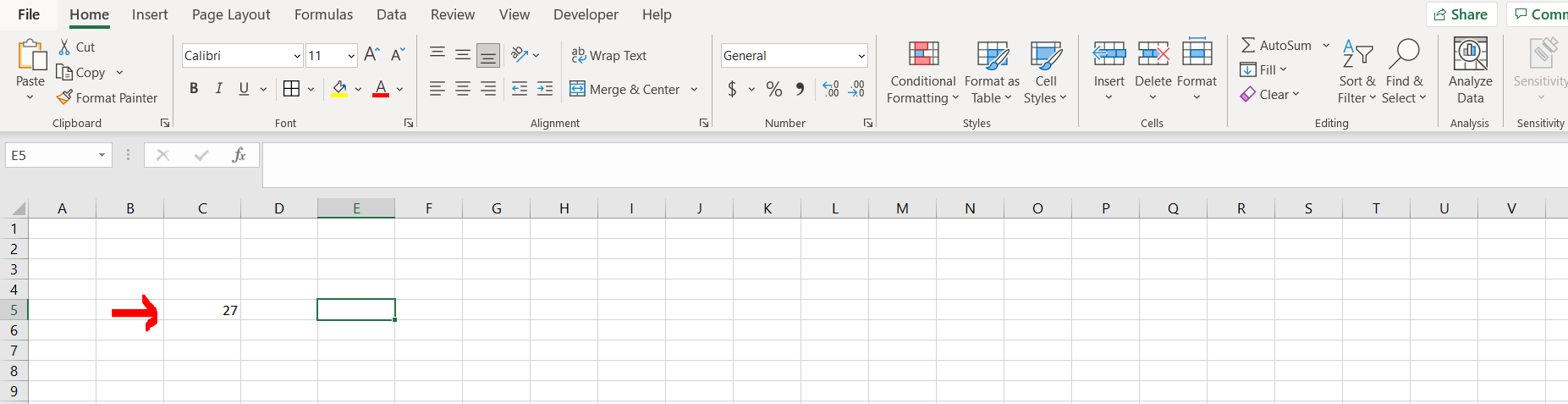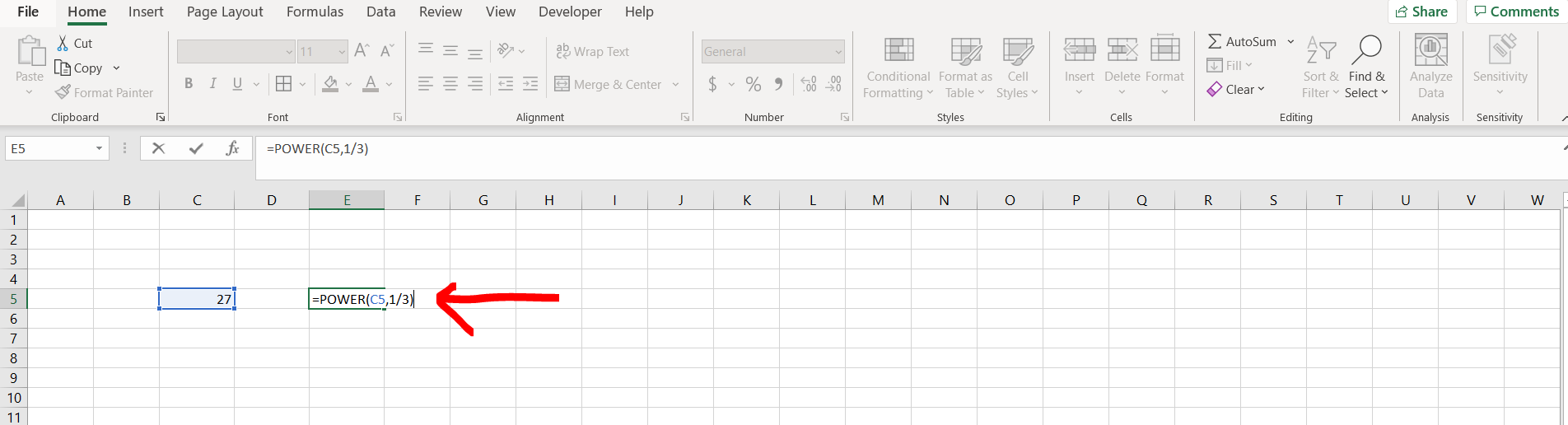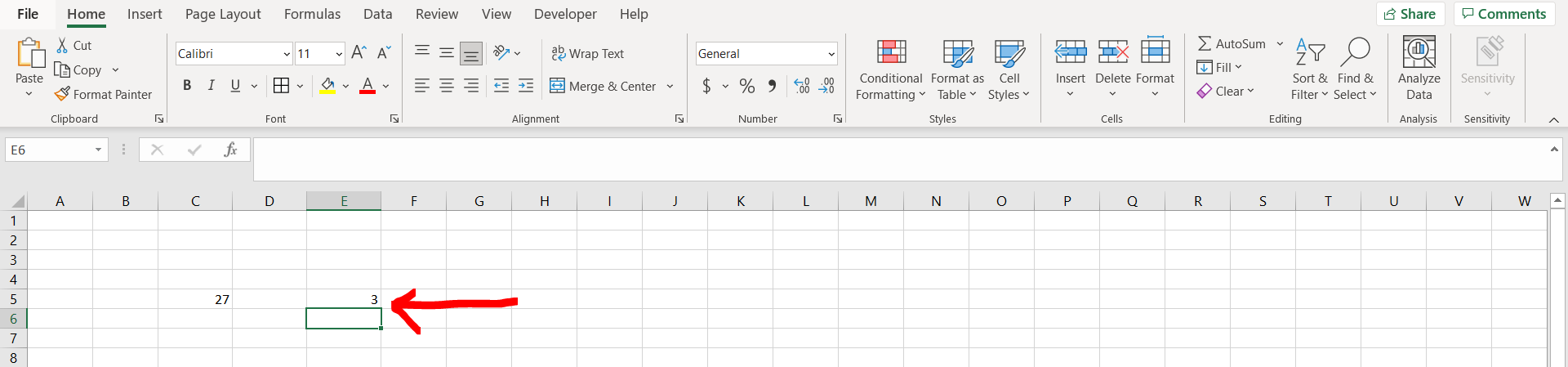# How to do cube root in ExcelExcel offers a very interesting way to do cube root. We can cater to this problem statement by using the “=power” formula. We can perform the below mentioned way to do cube root in excel:

### Step 1 – Excel workbook with a cube– Open the desired Excel workbook containing a cube which needs to be cube rooted

### Step 2 – Using the power formula– Now enter “=POWER(“ and then give reference to the cell which needs to be cube rooted followed by a comma, and then type “⅓”, and hit Enter.

### Step 3 – Cube root obtained– We can see that the cube root of the number has been obtained